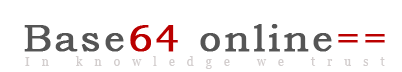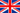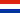Base64 decode | Decimal to binary | Hexadecimal converter | Hash generator | Colored QR Code
Cisco password cracker | Base64 to image | MAC address database| Online Device Explorer
Decode gzinflate base64 | IP Address | TCP-UDP Port check | Cisco docs

# Translate decimal to binary. Translating binary to decimal.

## How to translate binary to decimal manually?

The binary number system, or base 2, represents values with two chars, 0 and 1. Each digit is based on a power of number 2. We can use the table below to convert binary into decimal and decimal to binary. If the binary number is equal to 1 - the digit is "true", if it is 0 - the digit is "false". You do not need to write seven zeros to represent the binary value of 1, 00000001 is equal to 1 and 00100100 is equal to 00100100 or 36 in decimal.

 27=128 26=64 25=32 24=16 23=8 22=4 21=2 20=1 128 64 32 16 8 4 2 1 1 0 1 1 1 0 0 1

### Example.

Decimal value of 10111001 will be a resulting sum of "true" bits. 128 + 32 + 16 + 8 + 1 = 185.
To convert 10111001 back into decimal value, we must start by dividing 185 by first possible
value that is lower than 185.

185 / 128 = 1,
185 - 128 = 57.
Now we can divide 57 by 32,
57 / 32 = 1,
57 - 32 = 25.
25 / 16 = 1,
25 - 16 = 9.
9 / 8 = 1,
9 - 8 = 1.
1 / 1 = 1.

Now we got 128 = 1, 32 = 1, 16 = 1, 8 = 1, 1 = 1. But 64 = 0, 4 = 0 and 2 = 0.
If you put these values back into the table, you will get 10111001.

## Binary to decimal.

Online decode encode tools:

Base64 decode | Decimal to binary | Hexadecimal converter | Hash generator | QR Code
Cisco password cracker | Base64 to image | MAC address database | Online Device Explorer
Decode gzinflate base64 | IP Address | TCP-UDP Port check | Books | Hashes